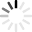Loading VESLSea level rise resulting from removing ice in Antarctica and/or Greenland.

Relative ice volume above flotation for Antarctica basins.

## Info

### Introduction

The simulation presented here uses NASA supercomputers and the Ice Sheet System Model framework, in which Sandia National Laboratories' statistical software is embedded in the Jet Propulsion Laboratory's ice sheet model, to run a range of 100-year warming scenarios for Antarctica. Presented results explore how the Antarctic Ice Sheet would change in ice volume above floatation in response to different climate forcing, using an uncertainty quantification sampling method. For various model forcing, including, snow accumulation, ice shelf basal melt rates, ice viscosity, and ice basal friction, we choose a spread of possible multipliers that represent variable change due to warming, ranging from present day values to those that could result from extreme climate warming.

Exploration of Antarctic Ice Sheet 100-year contribution to sea level rise and associated model uncertainties using the ISSM framework, The Cryosphere, 2018 contains further information about the methodology and datasets on which this simulation is based.

### Note

When varying a particular slider, a projected sea level change is computed based on a best fit curve, which is based on the model results. The closest result is then looked up, and these values are used to modify the Florida and Antarctica model simulations.

This scenario is hypothetical, and by no means fully representative of all the processes involved in both the evolution of polar ice sheets, and the way sea level rises differently in various parts of the world. It does not for example account for local subsidence of the ground, flooding, erosion of the shorelines, glacial isostatic rebound, negative feedbacks from the atmosphere, etc. It is, however, meant to assess the sensitivity of local sea level rise to melting of polar regions.

### Antarctica

• Antarctica surface elevation from Bamber et al., The Cryosphere, 2009, and Griggs and Bamber, The Cryosphere, 2009.
• Antarctica bedrock elevation from BEDMAP1+ -> merge of BEDMAP1 (Lythe et al, 2000) and bathymetry grids from Nitsche (LDEO) et al. 2007 and Tara Deen (BAS).
• Other Antarctica datasets found at the SeaRISE Present Day Antarctica webpage.

### Model Settings

• Antarctica mesh at resolution ranging from 20 to 60 km, split into 29 regions (27 regions as shown in Schlegel et al., 2018, plus Geographic Partition 4, which is divided into Pine Island Glacier, Thwaites Glacier, and the remaining area).
• Computation of SLR using volume above hydrostatic equilibrium and conversion to global mean sea level rise from global ocean surface area. New US coastline computed using simple comparison between surface height and new sea level.

### Calculating Height Scales for Antarctic Basins

On user interactions with the field sliders or PDF graph, the total sea level delta is estimated using a best fit curve to the sample data (field multipliers were plotted against total sea level data). Then, the sample with the total sea level delta closest to this estimate is retrieved. Each sample also includes the contribution of each of 29 Antarctic basins to the total sea level delta. Finally, these contributions are used to estimate the volumetric change as well as an appropriate height scale for each of the basins. The following pseudocode illustrates the procedure described above,

``````for (i = 0 to 29) {
basinContribution ← retrieve from sample

/**
* Overall ice equivalent volume change for basin
*
* NOTE:
*      - 361.9 Gt of ice to raise sea level by 1 mm
*      - Antarctic ice density: 0.8964 Gt / km^3
*/
basinVolumeDelta ← -basinContribution * 361.9 * Antarctic ice density; // km^3

// Calculate height scale for basin
basinHeightScales[i] ← basinVolumeDelta / basin surface area;
}

// Apply height scale to each vertex of mesh based on which basin it belongs to.
for (i = 0 to number of vertices in Antartica mesh) {
surface[i] ← surface[i] + basinHeightScales[i];
}``````

### How to Run the Simulation

When a variable is chosen by the user, all the possible contributions to global mean sea level rise from Antarctica are presented as a histogram (probability density function). When the user chooses a forcing multiplier for a particular variable, we highlight where results due to the change in forcing (variable field over the entire ice sheet multiplied by the multiplier) falls amongst all the possible 100-year outcomes, and we plot where sea level contribution comes from regionally in Antarctica. In addition, we present a plot showing what the coastal change would be for Florida (according to a simple "bathtub" model) for the chosen scenario.

### Feedback

If you have any questions or feedback, please send us an email.